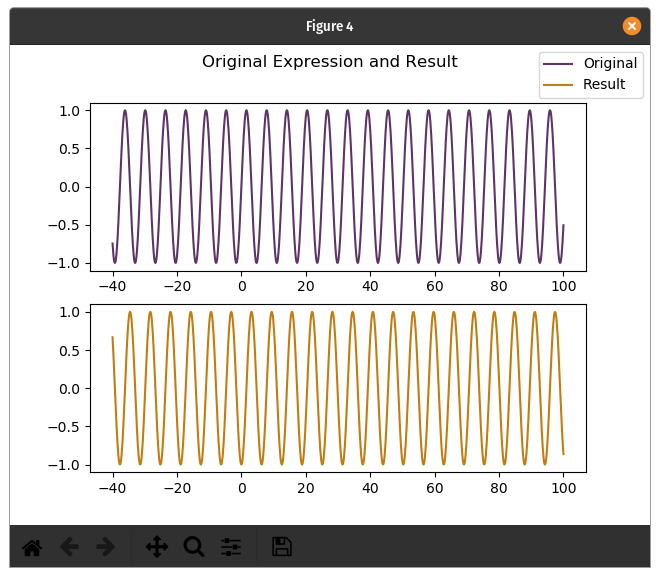# CalculusApplication to compute derivatives and integrals and plot the results

View the Project on GitHub carlos157oliveira/Calculus

# Calculus

Whether you are an undergraduate student or just a math geek, you will appreciate this application for calculating and plotting derivatives and integrals.

## Where to find

You can install the application from Flathub.

You will find other cool stuff there too!

## How to use

### Interface

When you open the application, you will see three fields:

• The main field is the expression you are performing the operation on;
• The second field is the variable with which the operation will be done. For example, use x if the operation is differentiate with respect to x (or integrate);
• The last is the chosen operation: differentiate or integrate.

### Simple operation

Put the function of interest in the main field.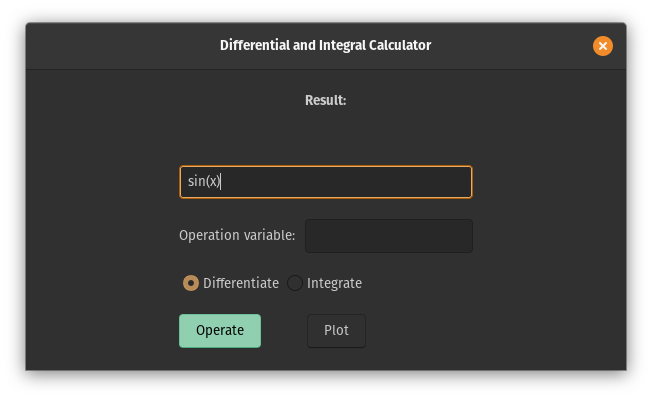Type in the variable in the second field (the operation will be done with it).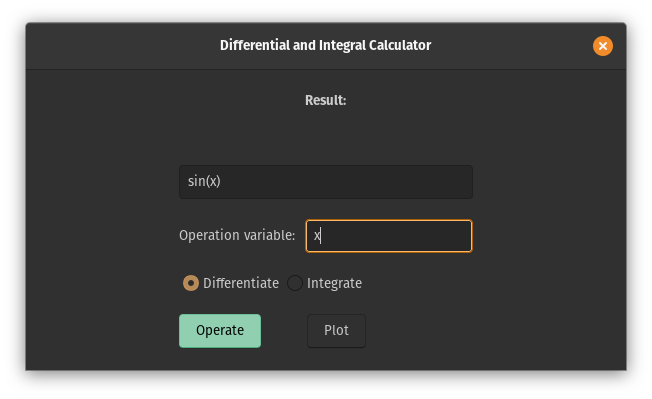Select between integration or differentiation (this is the default) and hit the Operate button!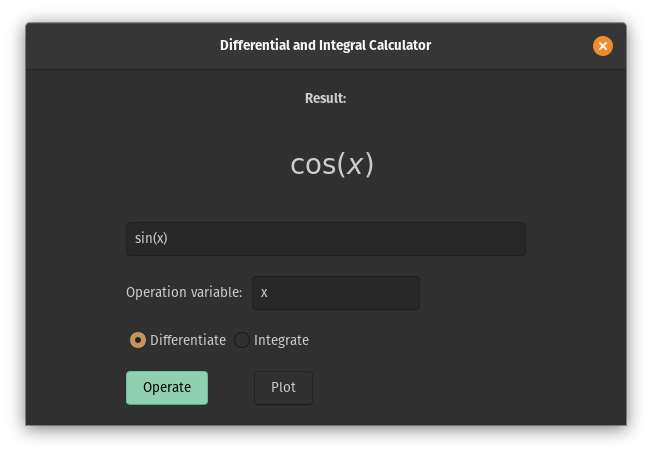If the operation variable is changed to y, then the differentiation of the last expression is zero because it is constant with respect to y.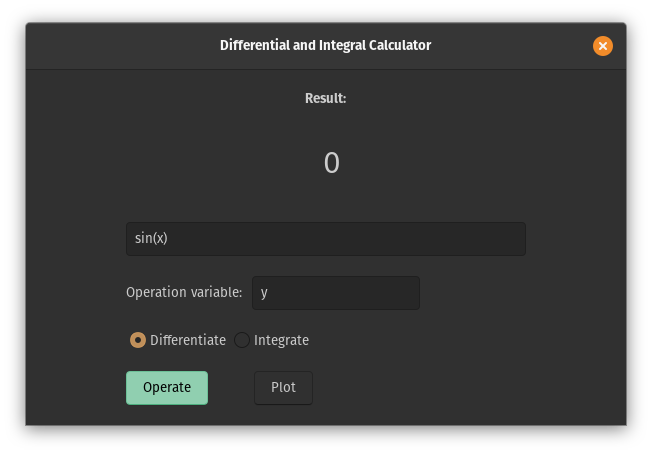### Gotchas

The back-end of the application is made with SymPy. There are some interesting things like:

• You must separate the variables with * (asterisk), don’t omit this symbol or it will be understood that the variable is named with more than one letter. Example: abc mean variable with name “abc” and not value a times b times c;
• The same apply to function. You can name a function with more than one letter, but remember to separate it from the expression using an algebraic operator;
• If the function is unknown to SymPy, it will display an integral or differentiation symbol and the plot functionality won’t be able to resolve the expression (image below show a unknown function xsin, which isn’t x*sin because the operator isn’t implicitly adopted);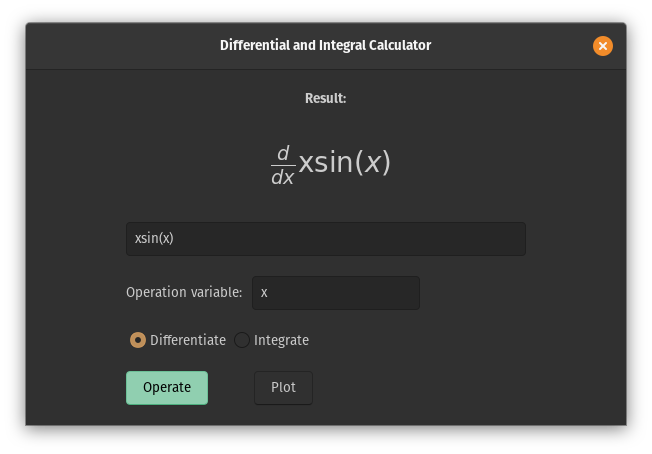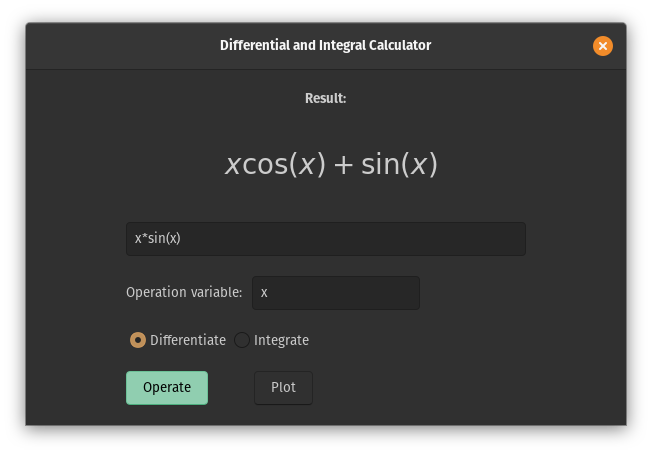• Sometimes, the result of an integral can be displayed using a function that has inside another integral.

### Plotting expressions

Not only can the application calculate derivatives and integrals, it can even plot the original expression and the displayed result! This only works with a univariable expression. If the expression is multivariable, consider substituting the variables that won’t be used with numerical fixed values.

After a result has been stored by the application, use the plot button.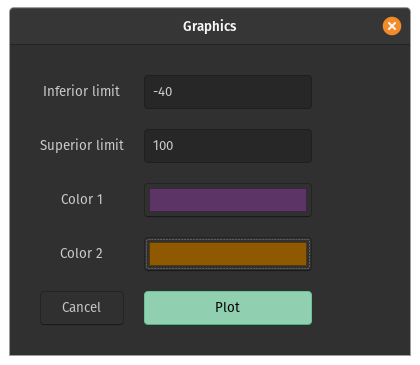Choose a range, the colors of the lines or leave the configuration as it is. Hit the Plot button and voilà. In the example below, the graphic of sinusoidal functions.